# excel的函数公式大全:如何学习excel函数？

SUM(参数1,参数2,……)

lookup向量形式时，要求查找区域中的值必须按升序排列，它的查找方法是二分法。

Vlookup要求查找对象，必须在查找区域中的第一列。

## 有些简单好用的excel函数公式？

1、查找重复内容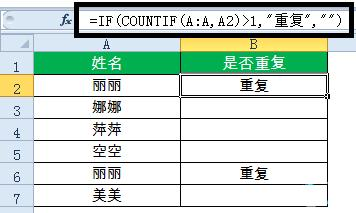=IF(COUNTIF(A:A,A2)>1,\”重复\”,\”\”)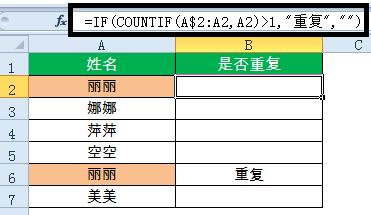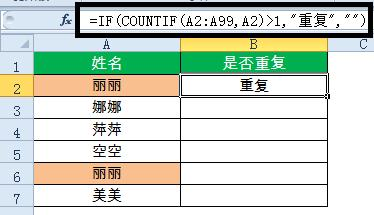2、重复内容首次出现时不提示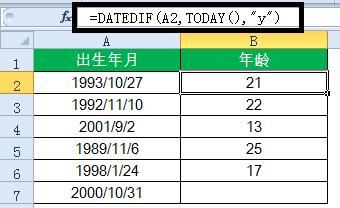=IF(COUNTIF(A\$2:A2,A2)>1,\”重复\”,\”\”)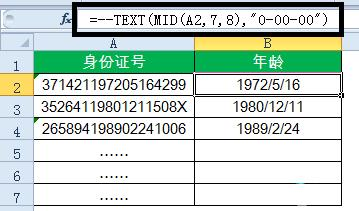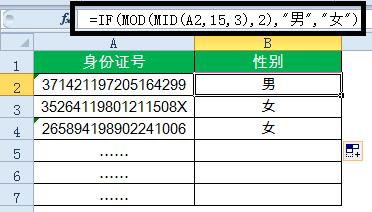3、重复内容首次出现时提示重复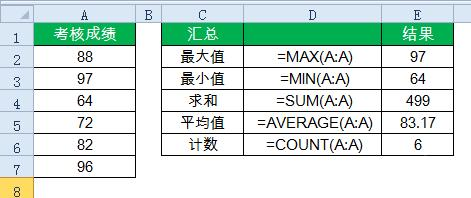=IF(COUNTIF(A2:A99,A2)>1,\”重复\”,\”\”)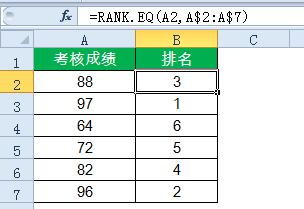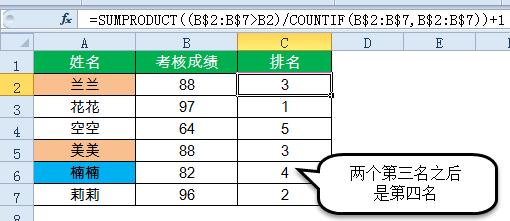4、根据出生年月计算年龄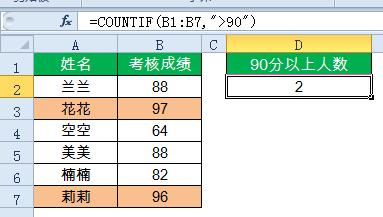=DATEDIF(A2,TODAY(),\”y\”)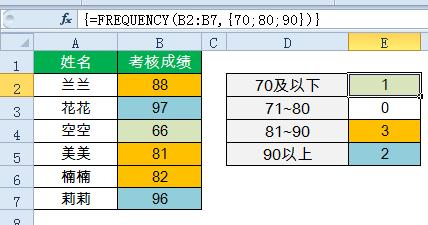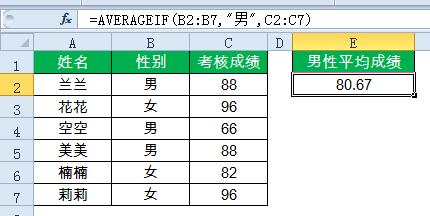5、根据身份证号码提取出生年月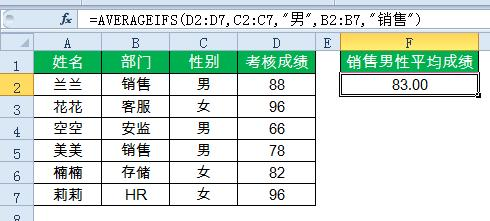=–TEXT(MID(A2,7,8),\”0-00-00\”)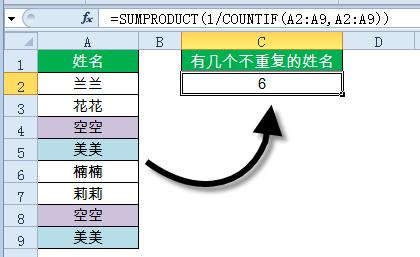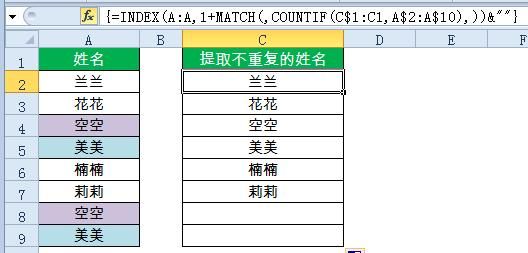6、根据身份证号码提取性别

=IF(MOD(MID(A2,15,3),2),\”男\”,\”女\”)

7、几个常用的汇总公式

A列求和：=SUM(A:A)

A列最小值：=MIN(A:A)

A列最大值：=MAX (A:A)

A列平均值：=AVERAGE(A:A)

A列数值个数：=COUNT(A:A)

8、成绩排名

=RANK.EQ(A2,A\$2:A\$7)

9、中国式排名（相同成绩不占用名次）

=SUMPRODUCT((B\$2:B\$7>B2)/COUNTIF(B\$2:B\$7,B\$2:B\$7))+1

10、90分以上的人数

=COUNTIF(B1:B7,\”>90\”)

11、各分数段的人数

=FREQUENCY(B2:B7,{70;80;90})

12、按条件统计平均值

=AVERAGEIF(B2:B7,\”男\”,C2:C7)

13、多条件统计平均值

=AVERAGEIFS(D2:D7,C2:C7,\”男\”,B2:B7,\”销售\”)

14、统计不重复的个数

=SUMPRODUCT(1/COUNTIF(A2:A9,A2:A9))

15、提取不重复的内容

C2单元格输入以下数组公式，按Ctrl+ Shift+Enter，向下复制。

=INDEX(A:A,1+MATCH(,COUNTIF(C\$1:C1,A\$2:A\$10),))&\”\”